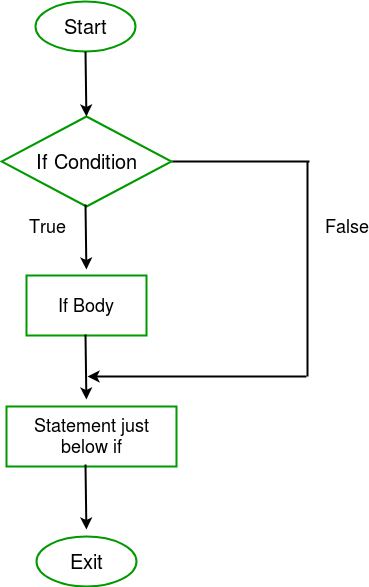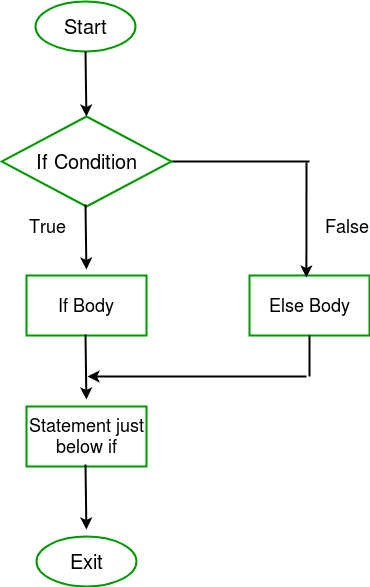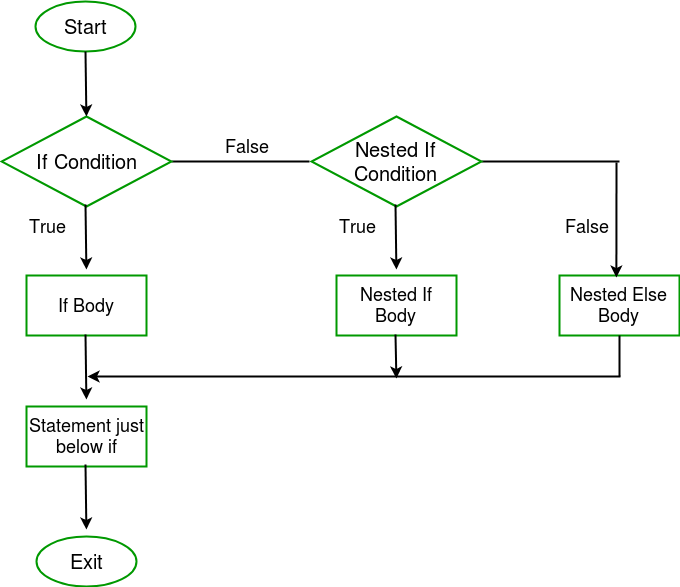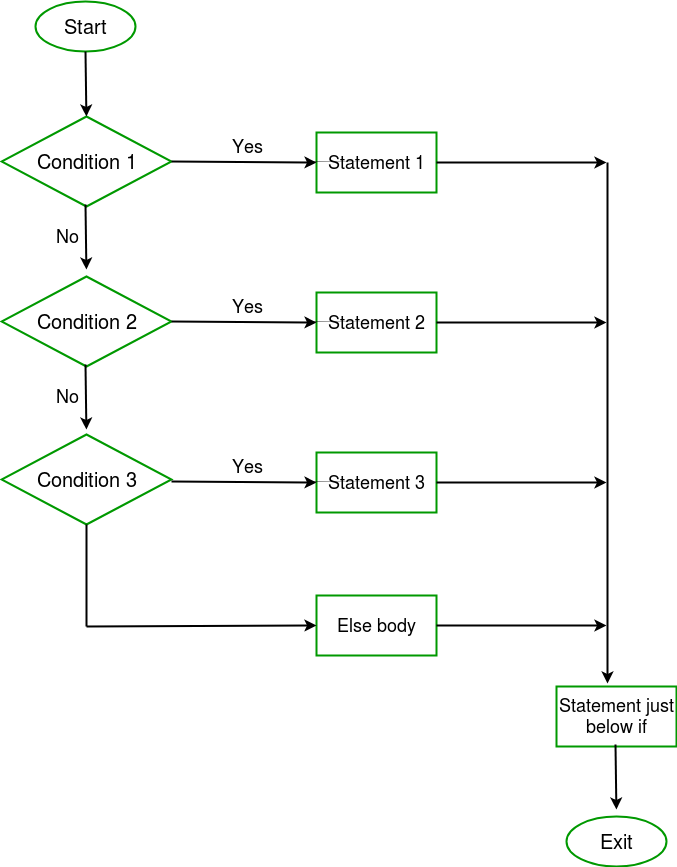×

Search anything:

# if else statement in C++

#### C++ Software Engineering if elseGet this book -> Problems on Array: For Interviews and Competitive Programming

There comes situations in real life when we need to make some decisions and based on these decisions, we decide what should we do next. Similar situations arises in programming also where we need to make some decisions and based on these decision we will execute the next block of code.

Decision making statements in programming languages decides the direction of flow of program execution. Decision making statements available in C++ are:

1)if statement
2)if..else statements
3)nested if statements

### if statement

if statement is the most simple decision making statement. It is used to decide whether a certain statement or block of statements will be executed or not i.e if a certain condition is true then a block of statement is executed otherwise not.Syntax :

``````            if(condition)
{
// Statements to execute if
// condition is true
}
``````

Here, condition after evaluation will be either true or false. if statement accepts boolean values – if the value is true then it will execute the block of statements below it otherwise not.
If we do not provide the curly braces ‘{‘ and ‘}’ after if( condition ) then by default if statement will consider the first immediately below statement to be inside its block.

// C++ program to illustrate If statement

``````#include<iostream>
using namespace std;

int main()
{
int i = 10;

if (i > 15)
{
cout<<"10 is less than 15";
}

cout<<"I am Not in if";
}
``````

Output :

``````I am Not in if
``````

As the condition present in the if statement is false. So, the block below the if statement is not executed.

### if- else

The if statement alone tells us that if a condition is true it will execute a block of statements and if the condition is false it won’t. But what if we want to do something else if the condition is false. Here comes the else statement. We can use the else statement with if statement to execute a block of code when the condition is false.Syntax :

``````                if (condition)
{
// Executes this block if
// condition is true
}
else
{
// Executes this block if
// condition is false
}
``````

### `C++` program to illustrate if-else statement

``````#include<iostream>
using namespace std;

int main()
{
int i = 20;

if (i < 15)
cout<<"i is smaller than 15";
else
cout<<"i is greater than 15";

return 0;
}
``````

Output :

``````i is greater than 15
``````

The block of code following the else statement is executed as the condition present in the if statement is false.

### nested-if

A nested if is an if statement that is the target of another if statement. Nested if statements means an if statement inside another if statement. Yes, C++ allows us to nest if statements within if statements. i.e, we can place an if statement inside another if statement.Syntax :

``````if (condition1)
{
// Executes when condition1 is true
if (condition2)
{
// Executes when condition2 is true
}
}
``````

### `C++` program to illustrate nested-if statement

``````int main()
{
int i = 10;

if (i == 10)
{
// First if statement
if (i < 15)
cout<<"i is smaller than 15";

// Nested - if statement
// Will only be executed if statement above
// it is true
if (i < 12)
cout<<"i is smaller than 12 too";
else
cout<<"i is greater than 15";
}

return 0;
}
``````

Output :

``````i is smaller than 15
i is smaller than 12 too
``````

Here, a user can decide among multiple options. The if statements are executed from the top down. As soon as one of the conditions controlling the if is true, the statement associated with that if is executed, and the rest of the ladder is bypassed. If none of the conditions is true, then the final else statement will be executed.Syntax :

``````            if (condition)
statement;
else if (condition)
statement;
.
.
else
statement;
``````

### `C++` program to illustrate if-else-if ladder

``````#include<iostream>
using namespace std;

int main()
{
int i = 20;

if (i == 10)
cout<<"i is 10";
else if (i == 15)
cout<<"i is 15";
else if (i == 20)
cout<<"i is 20";
else
cout<<"i is not present";
}
``````

Output :

``````i is 20
``````

### Question

Consider the following program:

``````#include <iostream>
#include <stdio.h>
int main()
{
if (!(std::cout << "hello"))
std::cout << "world";
else
std::cout << " else part";

return 0;
}
``````

#### What will be the output of the above program?

hello else part
hello world
world
else part
Since if-else works on the principle that if the condition provided in the if statement is true, if block is executed otherwise, else block will be executed. Since, std::cout<<"hello" returns a reference to std::cout, therefore, if condition becomes true and if block is executed.#### Harshita Sahai

Maintainer at OpenGenus | Previously Software Developer, Intern at OpenGenus (June to August 2019) | B.Tech in Information Technology from Guru Gobind Singh Indraprastha University (2017 to 2021)

if else statement in C++